# Meta-material research at NIT Trichy

New for April 2010! This work was contributed by Vidyalakshmi M. R., a Master of Science (by Research) student under the guidance of Dr. S. Raghavan, at NIT Trichy.

### Introduction

Metamaterials are artificially engineered materials which display negative permeability and permittivity over a certain range of frequency. First proposition of this was done by Prof. V.G. Veselago in 1968. Since realization of such materials was difficult at that time, there was very little progress in this field. But the first breakthrough was given by J.B. Pendry et al in 2000 by showing an array of two circular concentric rings and thin wire could exhibit the metamaterial property. The rings had a gap between them and hence the structure was named split ring resonator (SRR). We know that the lumped equivalent circuit of the SRR is an LC circuit. The various forms of SRR and complementary split ring resonator (CSRR) and their equivalent circuits are given in detail by Marques et al. Then in 2002, C. Caloz and T. Itoh gave the transmission line equivalent of metamaterials.

### Summary

A new structure of triangular split ring resonator with edge-coupling is proposed in . The structure consists of two equilateral concentric triangles made of metal with dielectric medium (Arlon Cu-clad substrate of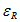=2.43) filled in the gaps. When the structure below is excited with a perpendicular magnetic field, the current flows through the rings gives rise to inductance and the capacitance arises out of the gap between the two rings.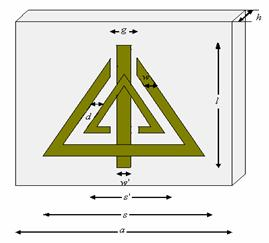Figure 1. Proposed structure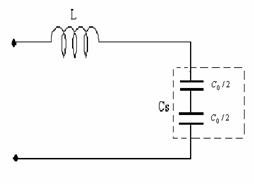z

Figure 2. Unit cell quivalent circuit

Hence the lumped equivalent circuit of this structure becomes an LC tank circuit. The advantage of this structure includes the reduction in size of the SRR and the wide range of resonant frequencies possible. The layout or the structure with the equivalent circuit and the Computer Aided Design model (CAD) design are presented. The Lorentz-Drude model had been derived for proving the negative values of effective permeability and permittivity.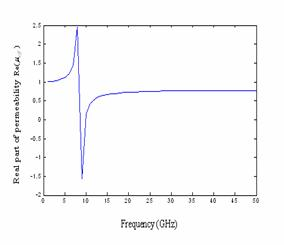Figure 3a. Real part of effective permeability versus frequency (GHz)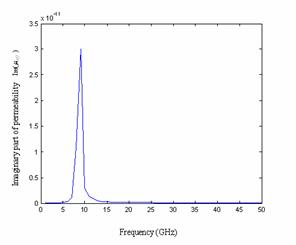Figure 3b. Imaginary part of effective permeability versus frequency (GHz)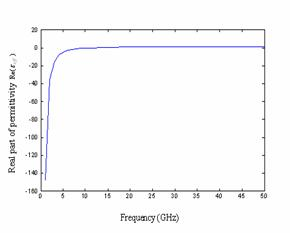Figure 4a. Real part of effective permittivity versus frequency (GHz)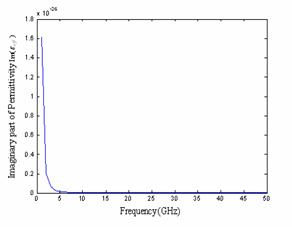Figure 4b. Imaginary part of effective permittivity versus frequency (GHz)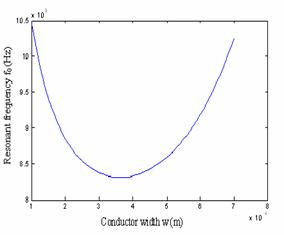Figure 5. Resonant frequency f0 (Hz) versus conductor width w (m)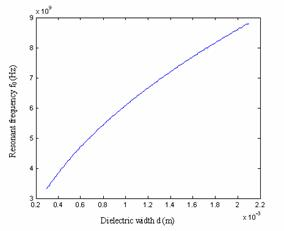Figure 6. Resonant frequency f0 (Hz) versus dielectric width d (m)

The variation of the resonant frequencies with respect to the conductor and dielectric width is also shown above in Figures 5 and 6. It also brings about the reduction in size to 46% as compared to the existing TSRR. There is also a good match with the values obtained theoretically and by simulation.

Another form of metamaterial is the planar version or the transmission line version known as left-handed transmission line (LH TL). But, realizing a pure LH TL was difficult because of the parasitic inductance and capacitance. So, we instead use a composite right left handed transmission line (CRLH TL). An accurate CAD model of the CRLH TL is proposed . It could be used for both balanced as well as unbalanced CRLH TL. The least-mean-square (LMS) error and the relative error plots between the theoretical and practical values are also presented below.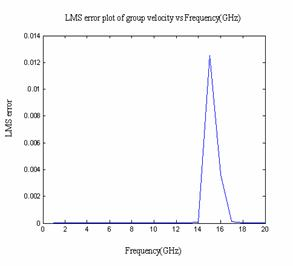Figure 7. Least-mean-square error of group velocity plotted versus frequency (GHz)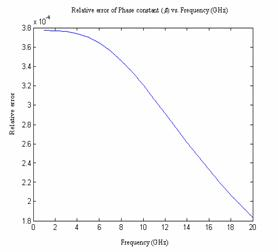Figure 8. Relative error of phase constant plotted versus frequency (GHz)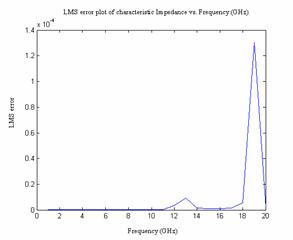Figure 9. Least-mean-square error of characteristic impedance plotted versus frequency (GHz)

It was observed that the phase velocity is negative and the group velocity is positive as it is the group velocity that deals with power flow. The CAD model accurately estimates all the secondary constants of the CRLH TL. Results shown in Figures 7-9 are in good agreement between the theoretical and obtained values.

Optimization before fabrication will help choose the right size and also would reduce fabrication cost. We shall present a work in which the size of the spilt ring resonator could be optimized for a particular resonant frequency by artificial neural networks and by genetic algorithm . Comparison of both the methods is also presented. For the ANN method, a feed-forward back propagation network and binary genetic algorithm type in genetic algorithm were used.

ANN performance curves are shown in Figure 11.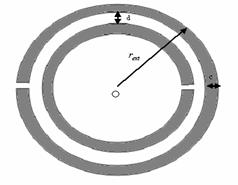Figure 10. Circular split ring resonator with the dimensions considered for optimization- external radius, conductor width and the dielectric width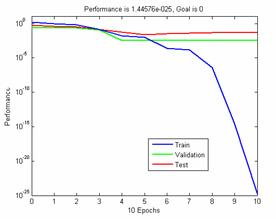Figure 11. Testing errors and training errors

TABLE I. Results of optimization with binary genetic algorithm for circular SRR

 Resonant Frequency (GHz) Conductor width c (mm) 5.15 7.6 0.254 0.2 0.179 0.2 5.5008 7.58 0.0001 0.0026

The fitness index value is taken to be absolute relative error between the desired and the calculated resonant frequencies. The external radius, the conductor width and the dielectric spacing between the two rings are taken as the parameters for optimization minimizing the error function to get the best dimensions for the given resonant frequency. In both cases as shown from Figure 11 and Table. I, error was found to well within the tolerable limits.

We have also presented an artificial neural network (ANN) model of a complementary circular split ring resonator . Taking advantage of Babinet's principle, we take a circular ring resonator and develop an ANN model for determination of its resonant frequency. Various algorithms of Levenberg-Marquardt scaled conjugate gradient and quasi newton methods are used to train the neural network and the results have been compared.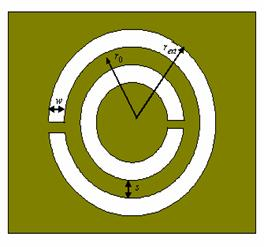Fig.12. Structure of circular complementary SRR with the dimensions of the slot-external, internal radius, width of the conductor and dielectric slot.

TABLE II. Resonant frequency calculated by all three algorithms for = 2.6mm, s=0.5mm and w=0.2mm

Algorithm Resonant
frequency (GHz)
Mean square error
Levenberg-Marquardt
algorithm
6.010 1.934 x 10-9
Scaled Conjugate
6.0099 1.00 x 10-8
Quasi Newton
algorithm
6.0077 5.2900 x 10-6

The ANN model for circular CSRR was developed using a three hidden layer feed forward back propagation network with the inputs as the dimensions of the CSRR and output being the resonant frequency using three training algorithms and was illustrated that Levenberg-Marquardt method of training algorithm was the best suited as it gave minimal error.

The three optimization techniques namely genetic algorithm (GA), artificial neural networks (ANN) and hybridization of GA-ANN is applied to the case of square split ring resonator in  and the obtained results are compared. In all the cases, the size of the split ring resonator is optimized in order to obtain minimum error in resonant frequency. There are many types of GA and ANN. Here, binary genetic algorithm and feed forward backpropagation ANN is chosen. A new method of hybridization is presented here which gives effective results.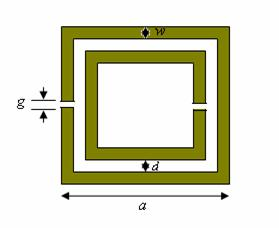Figure 13. Structure of square split ring resonator

### Results obtained through genetic algorithm (GA)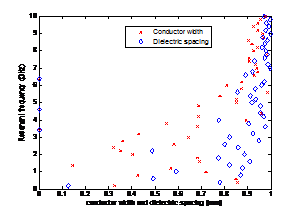Figure 14. Optimized values of conductor width and dielectric spacing of SSRR with respect to resonant frequency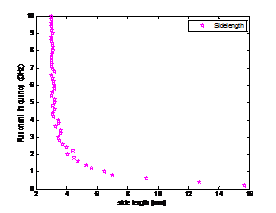Figure 15. Optimized value of side length of the SSRR with respect to resonant frequency

### Results obtained through artificial neural network (ANN)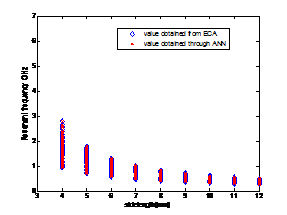Figure 16. Values of side length of the SSRR obtained at various frequencies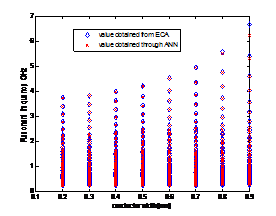Figure 17.Values of conductor width at various frequencies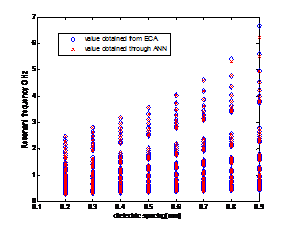Figure 18. Values of dielectric spacing between the rings

TABLE III. Dimensions of the SSRR obtained as a result of hybridization.

Resonant
frequency (GHz)
a (mm) w (mm) d (mm) Obtained resonant
frequency (GHz)
Absolute Error
1.0 6.2913 0.6323 0.6894 0.9995 0.0008
1.5 4.7410 0.3041 0.7167 1.4988 0.0008
2.0 4.4614 0.4114 0.9604 2.0006 0.0003
3.0 3.4066 0.4211 0.8575 3.0015 0.0005
4.0 3.2414 0.6182 0.9525 4.0176 0.0044
5.0 3.1144 0.7589 0.9375 5.0023 0.0005
6.0 3.1652 0.9085 0.9648 6.0047 0.0008

The error is also minimal in hybridization structure as compared to both the above techniques being used separately which are evident from the above Table.III

TABLE IV. Comparison between the optimization techniques presented.

Optimization
Technique
Accuracy Execution time Memory
requirement
BGA Good Large Large
ANN Less accurate Medium Less
Hybrid GA-ANN Best Large Medium

From Table IV, we understand the trade-off between the criteria of accuracy, execution time and memory requirement. Depending on the need, we are able to select the technique needed for the problem.A reconfigurable triangular split ring resonator using MEMS technology has also been proposed in . The gap in the outer ring of the triangular resonator can be adjusted with the help of control actuators for each side of the triangle thus making the resonant frequency shift. This can be used in various potential applications.ConclusionThus a new structure of triangular split ring resonator was proposed and the Lorentz-Drude model was also demonstrated along with the CAD model. The CRLH TL CAD model was also developed from the basic equations with minimal error. A series of optimization techniques of GA, ANN and its hybridization were applied to various resonators and its results were studied. A small proposal on reconfigurable MEMS split ring resonators was also made.

References

1.Vidyalakshmi. M.R and Dr. S. Raghavan," A CAD Model of Triangular Split Ring Resonator Based on Equivalent Circuit Approach", presented at IEEE AEMC 2009, Kolkata.

2. Vidyalakshmi. M.R and Dr. S. Raghavan, "CAD Model of a Composite Right Left Handed Transmission Line", ICMARS 2009, Jodhpur.

3. Vidyalakshmi. M.R and Dr. S. Raghavan,"Optimization of Circular Split Ring Resonator by Artificial Neural Networks and Genetic Algorithm", IEEE ICCCNT 2010, Karur. (Results awaited).

4. Vidyalakshmi. M.R and Dr. S. Raghavan, "A CAD Model of Complementary Circular Split Ring Resonator Using Artificial Neural Networks", ADVICE 2010, Chandigarh.

5. Vidyalakshmi. M.R and Dr. S. Raghavan, "Comparison of Optimization Techniques for Square Split Ring Resonator", IEEE ITSIM 2010. (Accepted).

6. Vidyalakshmi. M.R and Dr. S. Raghavan,"Reconfigurable Triangular split ring resonator with artificial neural network and Genetic algorithm analysis", Metamaterials 2010, Germany. (Results awaited).

Author : Vidyalakshmi M. R.